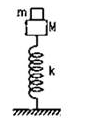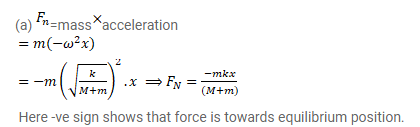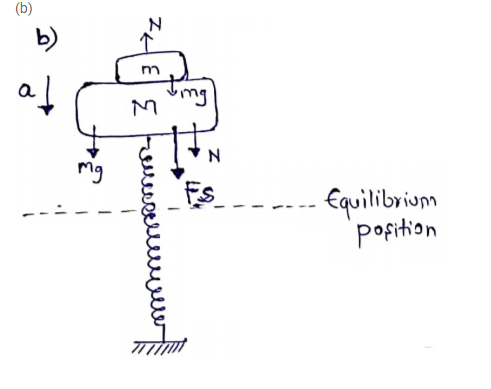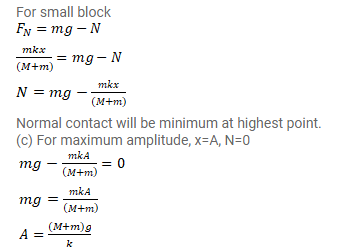# A small block of mass m is kept on a bigger block of mass M

Question:

A small block of mass $m$ is kept on a bigger block of mass $M$ which is attached to a vertical spring of spring constant $k$ as shown in figure. The system oscillates vertically.

(a) Find the resultant force on the smaller block when it is displaced through a distance $x$ above its equilibrium position.

(b) Find the normal force on the smaller block at this position. What is the force smallest in the magnitude?

(c) What can be the maximum amplitude with which the two blocks may oscillate together?Solution: